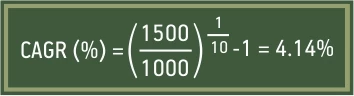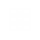• ## About Us

Aditya Birla Sun Life AMC Limited

# Why you should look for CAGR over absolute return?

Oct 22, 2019
6 mins | Views 8680Illustration: Last week a friend, Raj called me sounding confused. He was congratulating a co-worker for selling his house at double the buying price. However, his co-worker looked less happy. He shared with Raj, that they had just made 10% CAGR return. “Here I was thinking he made a 100% profit but according to him, it was barely 10%. Can you explain why there is such a huge difference,” he requested me.

In a nutshell, both absolute returns and CAGR are methods of return calculations. However, they differ in one important aspect that is that is ‘time’. To put things in perspective let us understand how absolute returns and CAGR returns are calculated.

Absolute return is the simplest form of return calculations. It only considers purchase value and sale value to calculate return.

Absolute return (%) = ((Current value of investment - Amount invested)/ Amount invested) * 100

So, if your investment amount is Rs. 1000 and the current value of investments is Rs. 1500. You have made 50% absolute returns.Now this is fantastic if you earned Rs. 500 profit in one year. However, if you earned it after investing the money for 10 years, it doesn't sound so nice, does it?

Thus, while investing for longer horizon along with the quantum of return it is also important to understand the pace at which your investment is growing every year – this is where CAGR (compounded annual growth rate) comes in picture.

Roughly, you can calculate annual return of your investment by dividing the total return by number of years.

Taking the earlier example,This means that annually, your investment grew at just 5% per annum

CAGR goes a step further. As the name suggests, CAGR also takes into account compounding while calculating returns. Compounding assumes that you reinvest your profits. As the profits will also generate returns, considering a period of 10 years, the final value of an investment growing at 5% CAGR will be higher than the final value of investment growing at 5% annually.

To summarise, CAGR is the rate at which the amount invested would need to grow to reach the current value assuming the profits earned are invested back every year.Let us take the earlier example,Thus, by considering time and the effect of compounding, CAGR helps you truly understand how your investment performed over long periods. It gives you a more accurate picture of the returns earned compared to absolute returns.

Moreover, CAGR makes it easier to compare two investments options when the holding period is different.

Say investment A is held for 3 years and gives an absolute return of 45% (amount invested Rs. 1000, current value Rs. 1450). Investment B meanwhile is held for 2 years and gives an absolute return of 35% (amount invested Rs. 1000, current value Rs. 1350).

Now if we just look at absolute returns then investment A makes more sense than investment B. However, if we calculate CAGR returns, then investment A gave CAGR returns of approximately 13% while investment B gave CAGR of approximately 16%. This means that investment B is growing at a faster pace than investment A. Thus, B may be a better option than A.

To summarise when we can consider investment horizon of more than a year it is better to look at CAGR returns because:

1. It gives a complete picture of gains made on your investment

2. It lets you easily compare two investments held for different periods

However, if the investment horizon is below one year then it is wiser to calculate absolute returns over CAGR returns as CAGR may inflate or shrink returns.

To illustrate, say your equity portfolio went up by 20% in a month. Then your absolute returns will be 20%. However, CAGR will assume that your returns will grow by 20% every month, which is unlikely.

Hence, while CAGR is the way to go if investment period is over a year, for shorter periods use absolute returns.

Mutual Fund investments are subject to market risks, read all scheme related documents carefully.

Rate this
Rate this Article
Leave a comment
Comment required
Name Required
Email ID required
Load More

##eAssistance

Give us a call on

1800 270 7000 within India

Get a call back

##Archives

Please Select Month
Please Select Year
Close
Hover to Zoom
BUY NOW